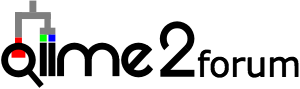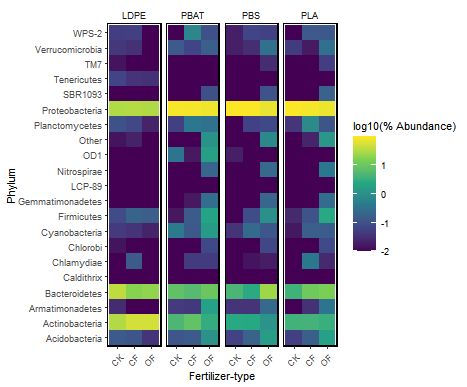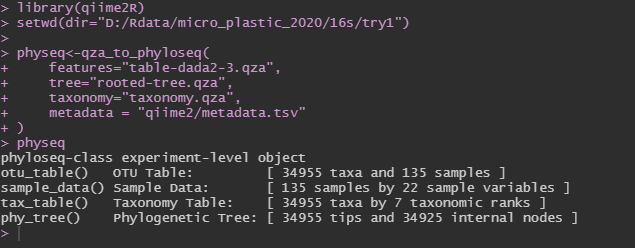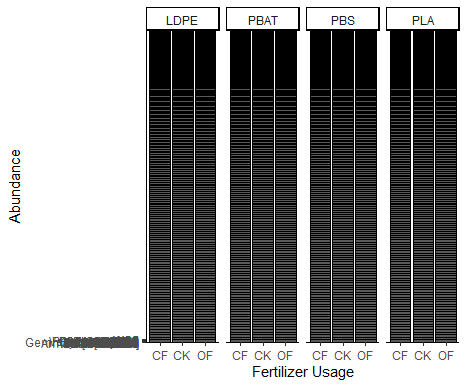# how to make a heatmap with phyloseq?

hello，I want to know how can I get the physeq？

hello sir. How can I get a phyloseq object through QIIME2r?

Hi colin @colinbrislawn,
Im making a heatmap now. Read your reply, I think use plot_heatmap is more easier than @jbisanz poseted, but I dont know, how to use it in the qiime2R. What‘s the “physeq” you said?
Is it this?
` physeq<-qza_to_phyloseq( features="table-dada2-3.qza", tree="rooted-tree.qza", taxonomy="taxonomy.qza", metadata = "qiime2/metadata.tsv" )`

Then I want to filter my sample,but it failed
physeq %>%
filter(`Source`==“Plastic”) %>%
tax_glom(“Phylum”) %>%
plot_heatmap() + facet_grid(~`Plastic-type`)
ggsave(“qiime2/image/heatmap.pdf”, height=4, width=20, device=“pdf”) # save a PDF 3 inches by 4 inches

what’ wrong?

I already used the command by @jbisanz to make a heat map, and I changed a little,
like this:

``````library(tidyverse)
library(qiime2R)
``````
``````setwd(dir="D:/Rdata/micro_plastic_2020/16s/try1")

SVs<-apply(SVs, 2, function(x) x/sum(x)*100) #convert to percent

TSVs<-SVs %>%
as.data.frame() %>%
rownames_to_column("Feature.ID") %>%
gather(-Feature.ID, key="SampleID", value="Abundance")
``````

# filter the data, extract top 30 specie

``````Table <-TSVs %>% left_join(metadata) %>% left_join(taxonomy) %>%  filter(`Source`=="Plastic")

TableToPlot<- group_by(Table, Feature.ID) %>%
summarize(MeanAbundance=mean(Abundance)) %>%
arrange(desc(MeanAbundance)) %>%
top_n(30, MeanAbundance) %>%
pull(Feature.ID) #extract only the names from the table

SVs %>%
as.data.frame() %>%
rownames_to_column("Feature.ID") %>%
gather(-Feature.ID, key="SampleID", value="Abundance") %>%
mutate(Feature.ID=if_else(Feature.ID %in% TableToPlot,  Feature.ID, "Other")) %>% #flag features to be collapsed
group_by(SampleID, Feature.ID) %>%
summarize(Abundance=sum(Abundance)) %>%
mutate(NormAbundance=log10(Abundance+0.01)) %>% # do a log10 transformation after adding a 0.01% pseudocount. Could also add 1 read before transformation to percent
left_join(taxonomy) %>%
filter(`Source`=="Plastic") %>%
mutate(Feature=paste(Feature.ID, Taxon)) %>%
mutate(Feature=gsub("[kpcofgs]__", "", Feature)) %>% # trim out leading text from taxonomy string %>%
mutate(`Fertilizer-type`=factor(`Fertilizer-type`, levels=c("CK","CF","OF"))) %>%
ggplot(aes(x=`Fertilizer-type`, y=Feature, fill=NormAbundance)) +
geom_tile() +
facet_grid(~`Plastic-type`, scales="free_x") +
theme_q2r() +
theme(axis.text.x=element_text(angle=45, hjust=1)) +
scale_fill_viridis_c(name="log10(% Abundance)")
ggsave("qiime2/image/heatmap.pdf", height=4, width=20, device="pdf") # save a PDF 3 inches by 4 inches
``````

Result

But,I want only display the “Phylum” level, So how should I do ? I just want filter my sample and display the “phylum” level ,with the abundance in heatmap.

Yu.

1 Like

@jbisanz
Hello,Sir
I drew the heat map using the following script .

setwd(dir=“D:/Rdata/micro_plastic_2020/16s/try1”)

#Phylum level
taxonomy_Phylum<-taxonomy %>% as.data.frame() %>%
rownames_to_column(“Feature.ID”) %>%
select(-c(“Class”,“Order”,“Family”,“Genus”,“Species”))
view(taxonomy_Phylum)
TSVs<-SVs %>%
as.data.frame() %>%
rownames_to_column(“Feature.ID”) %>%
gather(-Feature.ID, key=“SampleID”, value=“Abundance”)
#filter the sample according to metadata
FSVs <-TSVs %>%
left_join(taxonomy_Phylum) %>%
filter(`Source`==“Plastic”)

TableToPlot<- group_by(FSVs, Phylum) %>%
summarize(MeanAbundance=mean(Abundance)) %>%
arrange(desc(MeanAbundance)) %>%
top_n(20, MeanAbundance) %>%
pull(Phylum) #extract only the names from the table

FSVs %>%
#relative abundance
group_by(SampleID) %>%
mutate(SumAbundance=sum(Abundance)) %>%
group_by(SampleID, Phylum) %>%
mutate(Phylum_Abundance=sum(Abundance))%>%
mutate(Abundance=Phylum_Abundance/SumAbundance*100) %>%
mutate(Phylum=if_else(Phylum %in% TableToPlot,Phylum, “Other”)) %>% #flag features to be collapsed
mutate(NormAbundance=log10(Abundance+0.01)) %>%
#rank
mutate(`Fertilizer-type`=factor(`Fertilizer-type`, levels=c(“CK”,“CF”,“OF”))) %>%
#以“Plastic-type”
ggplot(aes(x=`Fertilizer-type`, y=Phylum, fill=NormAbundance)) +
geom_tile() +
facet_grid(~`Plastic-type`, scales=“free_x”) +
theme_q2r() +
theme(axis.text.x=element_text(angle=45, hjust=1)) +
scale_fill_viridis_c(name=“log10(% Abundance)”)
ggsave(“qiime2/image/heatmap.pdf”, height=4, width=20, device=“pdf”)but, is it correct to calculate the relative adbunce like this?

#average abundance
FSVs %>% group_by(SampleID) %>%
mutate(SumAbundance=sum(Abundance)) %>%
group_by(SampleID, Phylum) %>%
mutate(Phylum_Abundance=sum(Abundance))%>%
mutate(Abundance=Phylum_Abundance/SumAbundance*100) %>%
mutate(Phylum=if_else(Phylum %in% TableToPlot,Phylum, “Other”)) %>% #flag features to be collapsed
mutate(NormAbundance=log10(Abundance+0.01))

@jbisanz
Sir,I am a green hand in learning QIIME2 and R. So even if I have obtain the heatmap,but I’m not sure it is right or not. Can you help me check it?
My goal is use the data of some groups (plastic type) of samples not all my sample to make a heatmap in Phylum level. And , I want use a relative abundance and log normalized data.
Yu

Hi Yu,
Yes it looks like you are on the right track. You could also do it in less lines of codes by subsetting your input and using functions already in qiime2R with something like:

``````submeta<-subset(metadata, Source=="Plastic")
subsv<-SVs[,submeta\$SampleID]
tsum<-summarize_taxa(subsv, taxonomy)\$Phylum
taxa_heatmap(tsum, submeta, "Source", ntoplot=20)
``````

PS there could be typos in what I wrote so a little debugging might be necessary.

1 Like

Thank you!
I tried it.
But, a mistake happend.

``````library(qiime2R)
library(“ggplot2”)
library(phyloseq)
library(tidyverse)
``````
``````submeta<-subset(metadata, Source=="Plastic")
subSVs<-SVs[,submeta\$SampleID]
tsum<-summarize_taxa(subSVs,taxonomy)\$Phylum

taxa_heatmap(tsum, submeta, "Source", ntoplot=20）
ggsave("qiime2/image/heatmap.pdf", height=4, width=11, device="pdf")
``````

Error:unexpected input in “taxa_heatmap(tsum, submeta, “Source”, ntoplot=20）”

I modify the code several time, but it didnt work.

So,what’s wrong?

But I can use the code to make a barplot, like this

subSVs<-SVs[,submeta\$SampleID]
tsum<-summarize_taxa(subSVs,taxonomy)\$Phylum
taxa_barplot(tsum, submeta, “Plastic-type”, ntoplot=30)

How can I change my subgroup? such as this:because I have a subgroup"Fertilizer-type" under the group of “Plastic-type”.
I know how to change it in the original code,but i dont know in taxa_barplot.

In addition, how can I change the x axis to display the group and y axis display the log10?

@colinbrislawn
hi,colin.
I already used your measure to make a barplot, command like this:

library(qiime2R)

physeq<-qza_to_phyloseq(
tree=“rooted-tree.qza”,
taxonomy=“taxonomy.qza”,
)
physeqSo,I got a phyloseq object?

Then I use the phyloseq R package.
first, filterd the data.

sub=physeq %>% subset_samples(Source==“Plastic”) %>% subset_taxa(Kingdom==“Bacteria”)
sub %>%
tax_glom(“Phylum”) %>%
transform_sample_counts(function(x) 100*x/sum(x)) %>%
plot_bar(“Fertilizer.type”,“Phylum”) +
facet_grid(~`Plastic.type`) + theme_classic() +
xlab(“Fertilizer Usage”) +
ylab(“Abundance”) +
scale_fill_manual(values=c("#FF5A5F","#FFB400", “#007A87”))So,why it is black? and why do y axis lable overlape?

You are making good progress with Phyloseq!

So,I got a phyloseq object?

Yes! The function `qza_to_phyloseq()` returns a phyloseq object, which you have named `physeq`. You can now pass that phyloseq object to other functions.

Try this:

``````physeq %>%
subset_samples(Source=="Plastic") %>%
subset_taxa(Kingdom=="Bacteria") %>%
tax_glom("Phylum") %>%
transform_sample_counts(function(x) 100*x/sum(x)) %>%
plot_bar("Fertilizer.type","Phylum") +
facet_grid(Phylum~`Plastic.type`) + theme_classic() +
labs(x = "Fertilizer Usage", y = "Abundance")
``````

This is very similar to your code. The main change is to this function:
`facet_grid(Phylum~`Plastic.type`)`
I have added `Phylum` to the left of the `~` so that the y axis will be faceted by Phylum.

Let us know how the code works and what you try next.

Colin

I used the code above, it looked abit strange. How can I display top 20 phylum?

1 Like

Here’s how you can get the top 20

``````# Subset, then group taxa by phylum and transform to percent abundance.
physeq.phylum <- physeq %>%
subset_samples(Source=="Plastic") %>%
subset_taxa(Kingdom=="Bacteria") %>%
tax_glom("Phylum") %>%
transform_sample_counts(function(x) 100*x/sum(x))

# Get top 20 taxa
physeq.phylum.top <- prune_taxa(
names(sort(taxa_sums(physeq.phylum), TRUE))[0:20],
physeq.phylum)

# Plot
physeq.phylum.top %>%
plot_bar("Fertilizer.type","Phylum") +
facet_grid(Phylum~`Plastic.type`) + theme_classic() +
labs(x = "Fertilizer Usage", y = "Abundance")
``````

Hi，Colin，thank you ve ry much!
But,I want to display top20 phylum,and the rest phylum grouped together to “Others”.
and I want to know, how can I designate the order of the phylum name and the group of "Fertilizer.type?

Try this for the final plot:
(I’ve changed Phylum~`Plastic.type` to ~`Plastic.type`)

``````# Plot
physeq.phylum.top %>%
plot_bar("Fertilizer.type","Phylum") +
facet_grid(~`Plastic.type`) + theme_classic() +
labs(x = "Fertilizer Usage", y = "Abundance")
``````

Colin,thanks, I konw how to do. I put a plot as framework, then to chage labels.

1 Like

I’m sorry my suggestion did not work.

Colin,thanks, I konw how to do. I put a plot as framework, then to chage labels.

I’m glad you have a solution!